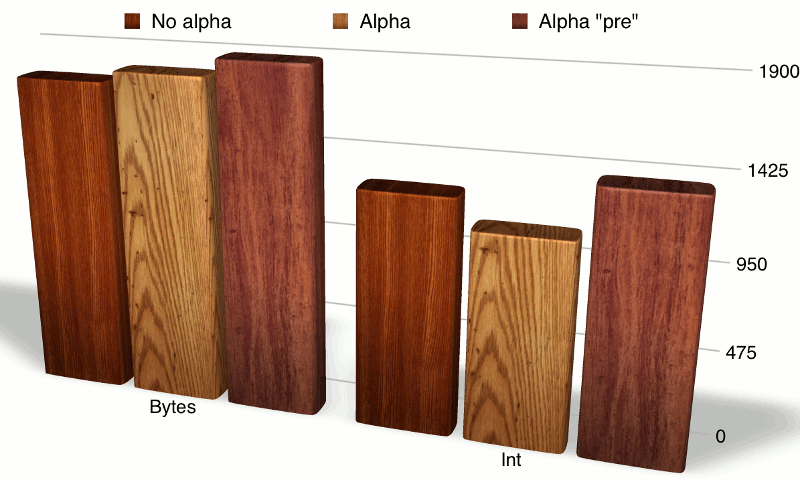# The performance of different BufferedImage types

On the previous pages, we saw how to create a BufferedImage in Java and how to set the pixels of an image using BufferedImage.setRGB().

We have mentioned that BufferedImages can be created with different types. On this page, I want to give a few performance indicators to help you in deciding which BufferedImage type to use.

If you have any comments, requests or other feedback relating to this information, you may leave feedback/questions/discussion here.Figure 1. Sample times for creating an image based on setting individual pixels via setRGB() using different buffered image types. Each data point is the mean time, in milliseconds, of 10 runs of 10 million iterations per run. In other words, each time represents the time in milliseconds to calculate and plot 10 million points on an image with the given type. The "3 Byte" measurement refers to TYPE_3BYTE_BGR; the "Int" measurement refers to the mean of TYPE_INT_RGB and TYPE_INT_BGR. The "Short" measurement is the mean of TYPE_USHORT_555_RGB and TYPE_USHORT_565_RGB types. These means are taken to simplify the graph as there is effectively no difference between the timings of the individual types that were averaged.

### Example: using setRGB() to create a fractal image (Iterated Function System)

As an initial indicator of performance, I present some results from a simple test program that creates a 512x512 fractal image of the so-called "Barnsley fern" using a technique called an Iterated Function System. If you're not familiar with this technique, the main things to be aware of are:

• the technique builds up an image by repeatedly calculating and then plotting points one at a time, up to some number of "iterations" (one point per iteration);
• from one point to a next, the calculation involves picking one of a set of transformations at random, then applying that transformation (consisting of a matrix multiplication and a vector addition) to the previous point;
• because there is an element of randomness in the relationship between one point and the previous point (specifically, we pick one of a number of transformations at random), points are not rendered in any particular order, hopefully minimising caching effects on our performance test.

I pick this test because I think it represents a "reasonable real-world case": we need to perform some small calculation before each point.Figure 2: setRGB() performance broken down by byte vs int representation and alpha status.

The results of this test are shown in Figure 1 above. Some key findings that come out of this comparison are:

• there is a large difference between performance of int vs byte representation, in this case with int representation performing better;
• the combination of integer representation and (non-premultiplied) presence of transparency performs best in this test, presumably because this is closest to the "native" format of bitmaps on this system and implementation;
• manipulating less data does not necessarily result in better performance: how close the data is to the native format seems more important (in particular, the "gray" format, with one byte per pixel, is actually among the worst performers);
• the choice is important: the difference between the best and worst case is almost double.

The interaction between byte/integer representation and presence of alpha (transparency) is shown more clearly in Figure 2. Here, we clearly see that integer representations as a whole perform better. As a whole, there is a slight performance hit for having alpha support, except for the "outlier" consisting of integer representation with non-premultiplied transparency.

The fact that premultiplied alpha performs worse may appear surprising: the whole point of multiplying alpha values with the other components is in principle a performance optimisation. Presumably on this system, alpha values are actually stored and manipulated in their "raw" state at a native level. You should at least take away from this that whether premultiplied alpha performs better or not should be measured, not assumed.